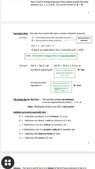# Sets being equal and equivalent

• B
• Osnel Jr
The author is saying that two sets can be equivalent if they have the same number of elements, even if those elements are not all distinct.f

#### Osnel Jr

TL;DR Summary
Kinda confused.
It says that sets that are equal ie having the same types of elements can also be equivalent, having the same number of elements when b has more distinct elements than a. Please explain

#### Attachments

•Screenshot_20210426-060710_Drive.jpg
39.1 KB · Views: 127
The author defines an equivalence relation between sets, i.e. he says that sets are equivalent, if there is a bijective mapping from one to the other. That is a map that uniquely maps all elements of one set to elements on the other.

In short: two sets are equivalent if they have the same number of elements. This is a relation between sets, because it sets two sets into relation to each other, namely, having the same number of elements or not.
Being an equivalence relation "##\sim##" means, it is
1. reflexive: ##M \sim M##
2. symmetric: ##M\sim N \Longrightarrow N\sim M##
3. transitive: ##M\sim N \text{ and }N\sim P \Longrightarrow M\sim P##
Having the same number of elements fulfills these conditions, so we can speak of an equivalence relation here.

The sets ##\{\text{ Soccer }, \text{ Golf }\}## and ##\{\text{ car }, \text{ bike }\}## are obviously not the same, but equivalent, because they have both two elements. We can map ##\text{ Soccer } \longrightarrow \text{ car }## and ##\text{ Golf }\longrightarrow \text{ bike }## and get a bijective map.

If we now compare ##\{\text{ Soccer }, \text{ Golf }\}## with ##\{\text{ Soccer }, \text{ Golf }\}##, then they are clearly equal. But they also have both two elements, which makes them equivalent. E.g. we can map ##\text{ Soccer } \longrightarrow \text{ Golf }## and ##\text{ Golf }\longrightarrow \text{ Soccer }## and get a bijective map. Equality is a special kind of equivalence relation. In our case, it is stronger, because not only the number of elements have to be equal, but the elements themselves have to be as well, in order to have equal sets.

•Klystron
Summary:: Kinda confused.

It says that sets that are equal ie having the same types of elements can also be equivalent, having the same number of elements when b has more distinct elements than a. Please explain
The author here appears to be working with what I would call "multi-sets" in which a particular element can appear more than once. So the multi-set {1,1} for instance has two elements. But it has only one "distinct element".

It is possible that the author is trying to lead you from a starting point talking about "multi-sets" to an end goal of talking about "sets" where the set elements are all distinct.

It is also possible that this will be done by introducing you to the notion of "equivalence classes" as @fresh_42 seems to suggest.

•Stephen Tashi
when b has more distinct elements than a. Please explain
In the text you quoted, the notation "##B = \{d,d,c,c,b,b,a,a\}##" is intended to denote a set with 4 distinct elements, For example, the notation "##d,d##" does not denote two distinct things both denoted by a "##d##". Instead it denotes the same thing ##d## listed twice. This notation convention is followed when writing elements of sets.

As @jbriggs444 pointed out, there is also concept called a "multiset". A multiset may contain several copies of the "same" thing. So if we were to consider "##B##" to be a multiset, it would contain 8 elements.

•jbriggs444
Cardinality and the listing of elements are the most basic properties of sets. Next along (may) come(s) an ordering of the elements

It says that sets that are equal ie having the same types of elements can also be equivalent, having the same number of elements when b has more distinct elements than a. Please explain
My guess is that the author is setting you up to consider sets that are not equal, but are equivalent, such as with certain infinite sets. For example, the set of natural numbers {1, 2, 3, ...} is equivalent to the set of positive even integers {2, 4, 6, ...}. This seems counterintuitive at first, since the first set has apparently more elements in it, but as long as a one-to-one mapping can be found from each set to the other, both sets have the same cardinality.

•Klystron